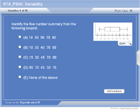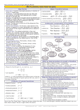How to Learn in 24 Hours?The Rapid Learning Movie

 Need Help? M-F: 9am-5pm(PST): Toll-Free: (877) RAPID-10 US Direct: (714) 692-2900 Int'l: 001-714-692-2900 24/7 Online Technical Support: The Rapid Support Center Secure Online Order:Got Questions? Frequently Asked Questions
 Need Proof? Testimonials by Our Users
 Trustlink is a Better Business Bureau Program. Rapid Learning Center is a fivr-star business. External TrustLink Reviews

 Rapid Learning Courses: MCAT in 24 Hours (2015-16) USMLE in 24 Hours (Boards) Chemistry in 24 Hours Biology in 24 Hours Physics in 24 Hours Mathematics in 24 Hours Psychology in 24 Hours SAT in 24 Hours ACT in 24 Hours AP in 24 Hours CLEP in 24 Hours DAT in 24 Hours (Dental) OAT in 24 Hours (Optometry) PCAT in 24 Hours (Pharmacy) Nursing Entrance Exams Certification in 24 Hours eBook - Survival Kits Audiobooks (MP3)

 Tell-A-Friend: Have friends taking science and math courses too? Tell them about our rapid learning system.Home »  Mathematics »  Introductory Statistics

Variability

 Topic Review on "Title": Key Formulas IQR = Q3 – Q1s2 = (1 / (n-1)) ∑(xi– x)2s = √s2 Variables Used Q1= 1st quartile Q3 = 3rd quartile M = Median s2 = variance s = standard deviation Q1 and Q3 To find 1st and 3rd quartiles:  Order the data, find n and median. Divide the data in half. Find the position of the middle value of each half. Determine the value represented by each middle position. Five Number Summary To find the five number summary: Order the data, find n and median. Find the 1st and 3rd quartiles. Order the five number summary according the symbols: Minimum Q1 M Q3 Maximum Standard Deviation When calculating the standard deviation, use a table to keep data separated and ordered. First, find the mean of the data set. Second, find the deviation from the mean of each observation. Third, square the deviations. Fourth, take the sum of the deviation squares. Fifth, find the variance. Sixth, take square root of variance to get standard deviation.

Rapid Study Kit for "Title":
 Flash Movie Flash Game Flash Card Core Concept Tutorial Problem Solving Drill Review Cheat Sheet"Title" Tutorial Summary : This tutorial describes what variability is and its properties. We will show you how to use specific measures of spread to interpret distributions, calculate and interpret quartiles, make a five number summary of data, construct a box plot of five number summary and calculate the standard deviation of a distribution. After we use the five number summary to make a box plot we will then know how to identify outliers that will greatly affect the variability of the distribution.

 Tutorial Features: Specific Tutorial Features: Step by step examples are shown to introduce variability. Examples as to how to interpret distributions, calculate and interpret quartiles are detailed in the tutorial. Details as to how to make a five number summary of data, construct a box plot of five number summary and calculate the standard deviation of a distribution are also presented with proper example in this tutorial. Series Features: Concept map showing inter-connections of new concepts in this tutorial and those previously introduced. Definition slides introduce terms as they are needed. Visual representation of concepts Animated examples—worked out step by step A concise summary is given at the conclusion of the tutorial.

 "Title" Topic List: Variability Quartiles Q1 Q3 Five number summary Boxplot Interquartile range Variance Standard deviation

See all 24 lessons in Introductory Statistics, including concept tutorials, problem drills and cheat sheets:
Teach Yourself Introductory Statistics Visually in 24 Hours